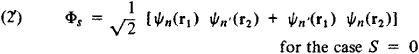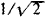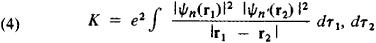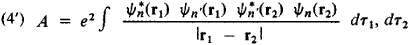# Exchange Interaction

(redirected from Exchange energy)

## Exchange interaction

A quantum-mechanical phenomenon that gives the energy of two elementary particles. Exchange effects arise for all kinds of elementary particles, but these effects were first introduced into physics in consideration of atomic structure and the energy of the electrons in an atom. In this context, they arise as a consequence of two facts: electrons are indistinguishable, and they obey the Pauli exclusion principle. See Elementary particle

Indistinguishability demands that the wave function describing two electrons either is unchanged or changes sign when the labels of the two electrons are exchanged (that is, it is either symmetric or antisymmetric to this exchange). In the case of electrons, the wave function is antisymmetric; this is a more precise statement of the Pauli exclusion principle. See Electron, Exclusion principle

When the spins of the two electrons are parallel the spatial part of the wave function is antisymmetric under exchange, and when they are opposed it is symmetric. The distribution in space of the two electrons is different in these two states, and so their mutual electrostatic energy is different. This difference in the electrostatic energy, called the exchange energy, appears as an interaction between the two electrons which depends on their relative orientation (although this dependence is incidental).

Exchange is the mechanism by which the electron spins in many magnetic materials are lined up parallel or opposed (for example, in α-iron and the ferrites). It is also the mechanism whereby the electron spins are parallel in the first excited state of the helium atom, and the spins of the two electrons are opposed in the carbon-carbon covalent bond. See Atomic structure and spectra, Electron configuration, Ferromagnetism, Quantum mechanics, Spin (quantum mechanics)

McGraw-Hill Concise Encyclopedia of Physics. © 2002 by The McGraw-Hill Companies, Inc.
The following article is from The Great Soviet Encyclopedia (1979). It might be outdated or ideologically biased.

## Exchange Interaction

a specific mutual effect of identical particles that is manifested in a certain special type of interaction. The exchange interaction is a purely quantum-mechanical effect that has no analogue in classical physics.

By virtue of the quantum-mechanical principle of the indistinguishability of identical particles, the wave function of a system must Have a definite symmetry with respect to the exchange of two identical particles, that is, the exchange of the coordinates and of the spins of the particles. For particles with integral spin—bosons—the wave function of a system does not change in such an exchange, that is, the wave function is symmetric; for particles with half-integral spins—fermions—the wave function changes sign, that is, it is antisymmetric. If the forces of interaction of the particles are independent of the particle spins, then the wave function of the system can be represented as the product of two functions, one of which depends only on the particle coordinates, and the other only on the particle spins. In this case, it follows from the principle of indistinguishability that the coordinate part of the wave function, which describes the motion of the particles in space, must have a definite symmetry with respect to the exchange of coordinates of identical particles; this symmetry depends on the symmetry of the spin wave function. The presence of such symmetry signifies that there is a certain coordination, or correlation, in the motion of identical particles. This correlation affects the energy of the system, even when there are no interactions between the particles due to forces of the usual type. Since an effect of one particle on another usually results from the action of certain forces between the particles, the mutual effect of identical particles that follows from the principle of indistinguishability is said to be the manifestation of a specific interaction—the exchange interaction.

The origin of the exchange interaction can be illustrated by using the helium atom as an example; this was first done by W. Heisenberg in 1926. Spin interactions in light atoms are weak; the wave function ψ of the two electrons in the helium atom therefore may be expressed as

(1) ψ = Φ (r1, r2) χ(s1, s2)

where Φ (r1, r2) is a function of the coordinates r1 and r2 of the electrons and χ(s1, s2) is a function of the components of the electron spins s1 and s1 in a certain direction. Since electrons are fermions, the total wave function Ψ must be antisymmetric. If the total spin S of the two electrons is equal to zero, in which case the spins are antiparallel and we have parahelium, then the spin function χ is antisymmetric with respect to the exchange of the spin variables and, consequently, the coordinate function Φ must be symmetric with respect to the exchange of the coordinates of the electrons. However, if the total spin of the system is equal to unity, in which case the spins are parallel and we have orthohelium, then the spin function is symmetric, and the coordinate function antisymmetric. If we designate the wave functions of the individual electrons in the helium atom as Ψn(r1) and Ψn(r2), where the subscripts n and n″ denote the set of quantum numbers defining the state of the electrons in the atom, and if we initially disregard the electron-electron interaction, then we can write the coordinate part of the wave function in the formThe factoris introduced to normalize the wave function. The mean distance between the electrons proves to be greater in the state with the antisymmetric coordinate function Φs; this can be seen from the fact that the probability

ǀψǀ2 = ǀΦaǀ2 ǀχsǀ2

of finding the electrons at the same point r1 = r2 is equal to 0 for the state Φa. The mean energy of the Coulomb interaction (repulsion) of the two electrons therefore proves to be less in the state Φa than in the state Φs. The correction for the energy of the system that is related to the electron-electron interaction is determined by perturbation theory and is equal to

(3) Eint = K + A

where the ± signs refer, respectively, to the symmetric coordinate state Φs and the antisymmetric coordinate state Φa and wherewhere dτ = dx dy dz is the volume element. The quantity K has a completely clear classical meaning and corresponds to the electrostatic interaction of two charged “clouds” with charge densities eǀΨn (r12 and eǀΨn″ (r22. The quantity A, called the exchange integral, may be interpreted as the electrostatic interaction of charged clouds with charge densities eΨ*n (r1n″ (r1) and [eΨ*nʹ; (r2n (r2), that is, when each of the electrons is located simultaneously in the states Ψn and Ψn″, a situation that is meaningless from the standpoint of classical physics. It follows from (3) that the total energies of parahelium and orthohelium with the electrons in analogous states differ by the quantity 2A. Thus, although the spin interaction itself is small and is disregarded, the indistinguishability of the two electrons in the helium atom leads to a situation in which the energy of the system proves to depend on the total spin of the system, as if an additional interaction, the exchange interaction, existed between the particles. It is obvious that in this case the exchange interaction appears as a part of the Coulomb interaction of the electrons and enters in explicit form in the approximate consideration of the quantum-mechanical system in which the wave function of the whole system is expressed in terms of the wave functions of the individual particles, in particular, in the Har-tree-Fock approximation.

The exchange interaction makes its presence felt whenever the wave functions of the individual particles of a system “overlap,” that is, when there exist regions of space in which particles in different states of motion can be located with nonnegligible probability. This can be seen from the expression for the exchange integral A: if the degree of overlapping of the states Ψ*n(r) and Ψ*n″(r) is insignificant, then the magnitude of A is very small.

It follows from the principle of indistinguishability that the exchange interaction arises in a system of identical particles even if interactions of the particles by forces of the usual type may be disregarded, that is, even in an ideal gas of identical particles. The exchange interaction first makes its presence felt when the average distance between particles becomes comparable with or is less than the de Broglie wavelength that corresponds to the average velocity of the particles. In this case, the nature of the exchange interaction differs for fermions and bosons. For fermions, the exchange interaction is a consequence of the Pauli exclusion principle, which hinders the approach of identical particles with spins in the same direction and is in effect manifested as a mutual repulsion of these particles at distances of the order of or less than the de Broglie wavelength; this exchange interaction is entirely responsible for the energy of a degenerate gas of fermions (a Fermi gas) being nonzero. By contrast, in a system of identical bosons, the exchange interaction resembles a mutual attraction of the particles. In these cases, systems consisting of a large number of identical particles are investigated on the basis of quantum statistics—Fermi-Dirac statistics for fermions and Bose-Einstein statistics for bosons.

If interacting identical particles are located in an external field, such as the Coulomb field of a nucleus, then the existence of a definite symmetry of the wave function and, correspondingly, the existence of a certain correlation among the motions of the particles affect the energy of the particles in this field. This too is an exchange effect. Such an exchange interaction usually, in an atom, molecule, or crystal, makes a contribution of opposite sign to that of the exchange interaction of the particles among themselves. Therefore, the total exchange effect can either decrease or increase the total energy of interaction in the system. Whether a state with fermions—particularly electrons—having parallel spins is energetically favorable or not depends on the relative magnitudes of these contributions. For example, in a ferromagnet—just as in the helium atom examined above—the state in which the spins of the electrons in unfilled shells of neighboring atoms are parallel has a lower energy; in this case, spontaneous magnetization occurs as a result of the exchange interaction. By contrast, in molecules with a covalent chemical bond, such as the H2 molecule, the state in which the spins of the valence electrons of the bonding atoms are antiparallel is energetically favorable.

The exchange interaction thus explains certain features of atomic and molecular spectra, the chemical bond in molecules, ferromagnetism and antiferromagnetism, and also other specific phenomena in systems of identical particles.

The term “exchange interaction” is also used to denote interaction forces that are not due to the indistinguishability of the particles but that lead to an “exchange” of certain characteristics between particles. For example, among the different types of nuclear forces, there are some forces by means of which nucleons—protons and neutrons—of a nucleus “exchange” coordinates, directions of spin, and electric charge. Such forces are called exchange forces and result from the ability of nucleons to exchange various types of mesons; such exchanges transfer charge, spin, and other quantum characteristics from one nucleon to another.

### REFERENCES

Blokhintsev, D. I. Osnovy kvantovoi mekhaniki, 3rd ed. Moscow, 1961.
Gombás, P. Statisticheskaia teoriia atoma i ee primeneniia. Moscow, 1951. (Translated from German.)
Vonsovskii, S. V., and Ia. S. Shur. Ferromagnetizm. Moscow-Leningrad, 1948.
Davydov, A. S. Teoriia atomnogo iadra. Moscow, 1958.

D. A. KIRZHNITS and S. S. GERSHTEIN

The Great Soviet Encyclopedia, 3rd Edition (1970-1979). © 2010 The Gale Group, Inc. All rights reserved.

## exchange interaction

[iks′chānj ‚int·ə′rak·shən]
(quantum mechanics)
An interaction represented by a potential involving exchange of space or spin coordinates, or both, of the particles involved; can be visualized physically in terms of exchange of particles.
Any interaction which can be looked upon as due to exchange of particles.
McGraw-Hill Dictionary of Scientific & Technical Terms, 6E, Copyright © 2003 by The McGraw-Hill Companies, Inc.
References in periodicals archive ?
The two sides also agreed to complete the technical and economic studies required for the implementation of the second phase, which relate to the interconnectivity and mutual exchange between the two countries, which requires the completion of the internal energy transmission networks between the two countries and strengthening the exchange capacity, it is expected to complete this stage in 2025, to be a promising market for Iraq to exchange energy with neighboring countries./ End
Although the Egyptian Electricity Transmission Company (EETC) has completed linking the extension of the Toshka substation to the electric interconnection project with Sudan and its willingness to export and exchange energy with the neighbouring country, but the fate of the project remains unknown due to the ongoing events and political unrest in Khartoum.
Zawati highlighted investment opportunities in the natural recourses sector and the Kingdom's expertise in renewable energy, stressing the importance of the joint Jordanian-Czech committee meetings currently held in Amman to bolster cooperation and exchange energy expertise.
In the case of disruptions, Member States should be able to exchange energy supplies without problems.
The Larnaca project will make use of Flowserve Calder Dual Work Exchange Energy Recovery units together with Flowserve DMX pumps in the high pressure feed service.
Turkish Electricity Transmission Company (TEIAS) will be able to exchange energy with European electricity market thanks to this technology.
Let V be the interaction between electrons (which is mainly electrostatic), and define the first-order approximate energy as the energy associated with a given configuration (the sum of the eigenvalue of the equation for independent electrons, the exchange energy, and the diagonal term of V).
"How these two fields exchange energy with each other constitutes the basic physics of turbulent reacting flows."
For example, in Kalundborg, Denmark's industrial district, firms exchange energy, water and materials to reduce the impact of environmental pollutants.
This surprising sleight of hand suggests that molecules dissolved in water--for example, many biological molecules--may exchange energy via a previously unknown fast track through the solvent.
Al-Fahdawi pointed out that "Iraq is seeking to exchange energy with Jordan under the Eight Link Agreement." For his part, the Jordanian ambassador pledged "to activate all agreements and economic exchanges between Jordan and Iraq."/ End
This will allow both countries to secure and exchange energy. During non-peak hours, both countries will exchange excess energy on a commercial basis.

Site: Follow: Share:
Open / Close